Students can access the CBSE Sample Papers for Class 10 Maths Standard with Solutions and marking scheme Set 2 will help students in understanding the difficulty level of the exam.

## CBSE Sample Papers for Class 10 Maths Standard Set 2 with Solutions

Time: 3 Hours
Maximum Marks: 80

General Instructions:

1. This question paper contains two parts, A and B.
2. Both Part A and Part B have internal choices.

Part-A:
1. It consists of two sections, I and II.
2. Section I has 16 questions of 1 mark each. Internal choice is provided in 5 questions.
3. Section II has 4 questions on case study. Each case study has 5 case-based sub-parts. An examinee is to attempt any 4 out of 5 sub-parts.

Part-B:
1. It consists of three sections III, IV and V.
2. In section III, Question Nos. 21 to 26 are Very Short Answer Type questions of 2 marks each.
3. In section IV, Question Nos. 27 to 33 are Short Answer Type questions of 3 marks each.
4. In section V, Question Nos. 34 to 36 are Long Answer Type questions of 5 marks each.
5. Internal choice is provided in 2 questions of 2 marks, 2 questions of 3 marks and 1 question of 5 marks.

Part – A
Section-I

Section 1 has 16 questions of 1 mark each. Internal choice is provided in 5 questions.

Question 1.
If xy = 180 and HCF(x, y) = 3, then find the LCM(x, y).
OR
The decimal representation of will terminate after how many decimal places?
Solution :
(LCM)(3)= 180
LCM = 60
OR
Four decimal places.

Question 2.
If the sum of the zeroes of the quadratic polynomial 3×2 – kx + 6 is 3, then find the value of k.
Solution :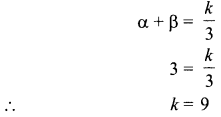Question 3.
For what value of k, the pair of linear equations 3x + y = 3 and 6.x + ky = 8 does not have a solution.
Solution :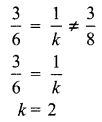Question 4.
If 3 chairs and 1 table costs ₹ 1500 and 6 chairs and 1 table costs ₹ 2400, form linear equations to represent this situation.
Solution :
Let the cost of 1 chair = ₹ x
And the cost of 1 table = ₹ y
3 x + y= 1500
6x + y = 2400

Question 5.
Which term of the AP 27, 24, 21, is zero?
OR
In an Arithmetic Progression, if d = -4, n = 7, an = 4, then find a.
Solution :
an = a + (n – 1) d
0= 27 + (n-1)(-3)
30= 3n
n= 10
10th term.
OR
an= a + (n — 1 )d
4 = a + 6 X (—4)
a = 28

Question 6.
For what values of k, the equation 9x2 + 6kx + 4 = 0 has equal roots?
Solution :
9x2 +6kx + 4 = 0
(6k)2-4 x 9 x 4 = 0
36k2 = 144
⇒ k= 4
k = ±2

Question 7.
Find the roots of the equation x2 + 1x + 10 = 0.
OR
For what value(s) of ‘a’ quadratic equation 3ax2 – 6x + 1 = 0 has no real roots?
Solution :
x2 + 7x+ 10= 0
x2 + 5x + 2x+ 10= 0
(x + 5)(x + 2) = 0
x = -5, x = -2
OR
3ax2 – 6x + 1 = 0
(-6)2– 4(3a)(1) < 0
12a > 36
⇒ a > 3

Question 8.
If PQ = 28 cm, then find the perimeter of ΔPLM.PQ = PT

PL + LQ= PM + MT
PL + LN = PM + MN
Perimeter (ΔPLM) = PL + LM + PM
= PL + LN + MN + PM = 2 (PL + LN) = 2(PL + LQ)
= 2 x 28 = 56 cm

Question 9.
If two tangents inclined at 60° are drawn to a circle of radius 3 cm, then find length of each tangent.
OR
PQ is a tangent to a circle with centre O at point P. If ∠OPQ is an isosceles triangle, then find ∠OQP.Question 10.
In the AABC, D and E are points on side AB and AC respectively such that DE || BC. If
AE = 2 cm, AD = 3 cm and BD = 4.5 cm, then find CE.Question 11.
In the figure, if B1, B2, B3,…. and A1, A2, A3,….. have been marked at equal distances. In what ratio C divides AB?8:5

Question 12.
sin A + cos B =1, A = 30° and B is an acute angle, then find the value of B.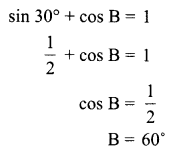Question 13.
If x = 2 sin2 θ and y = 2 cos2 θ + 1, then find x + y
x + y = 2sin2
0 + 2 cos2 9 + 1
= 2(sin2 0 + cos2 0) + 1
= 3

Question 14.
In a circle of diameter 42 cm,if an arc subtends an angle of 60° at the centre where n = then what will be the length of arc?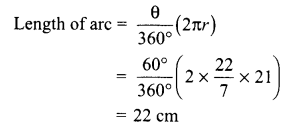Question 15.
12 solid spheres of the same radii are made by melting a solid metallic cylinder of base diameter 2 cm and height 16 cm. Find the diameter of the each sphere.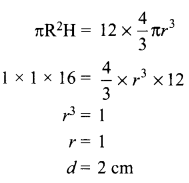Question 16.
Find the probability of getting a doublet in a throw of a pair of dice.
OR
Find the probability of getting a black queen when a card is drawn at random from a well- shuffled pack of 52 cards.Section-II

Case Study based questions are compulsory. Attempt any four sub-parts of each question. Each sub-part carries 1 mark.

Case Study Based-1 Sun Room

Question 17.
The diagrams show the plans for a sun room. It will be built onto the wall of a house. The four walls of the sun room are square clear glass panels. The roof is made using four clear glass panels, trapezium in shape, all the same size

• one tinted glass panel, half a regular octagon in shape.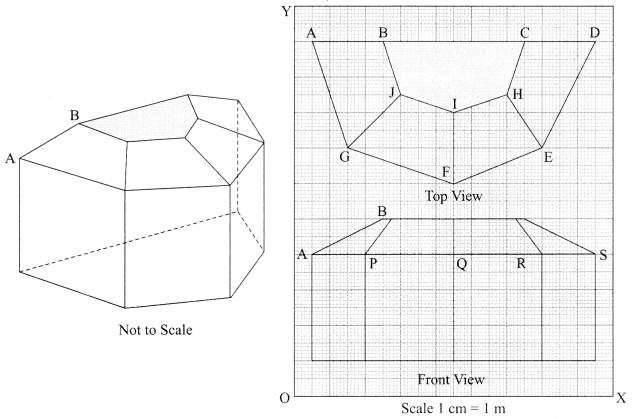Refer to Top View
(a) Find the mid-point of the segment joining the points J (6, 17) and I (9, 16).
(i) $$\left(\frac{33}{2}, \frac{15}{2}\right)p$$
(ii) $$\left(\frac{3}{2}, \frac{1}{2}\right)$$
(iii) $$\left(\frac{15}{2}, \frac{33}{2}\right)$$
(iv) $$\left(\frac{1}{2}, \frac{3}{2}\right)$$
Solution:
(iii) $$\left(\frac{15}{2}, \frac{33}{2}\right)$$

Refer to Front View
(b) The distance of the point P from the y-axis is
(i) 4
(ii) 15
(iii) 19
(iv) 25
Solution:
(i) 4

Refer to Front View
(c) The distance between the points A and S is
(i) 4
(ii) 8
(iii) 16
(iv) 20
Solution:
(iii) 16

Refer to Front View
(d) Find the coordinates of the point which divides the line segment joining the points A and B in the ratio 1 : 3 internally.
(i) (8.5, 2.0)
(ii) (2.0, 9.5)
(iii) (3.0, 7.5)
(iv) (2.0, 8.5)
Solution:
(iv) (2.0, 8.5)

Refer to Front View
(e) If a point (x,v) is equidistant from the Q(9. 8) and S( 17, 8), then 1
(i) x + y = 13
(ii) x – 13 = 0
(iii) x – 13 = 0
(iv) x – y – 13
Solution:
(ii) x – 13 = 0

Case Study Based-2

Question 18.
Scale Factor and Similarity
Scale FactorSolution :
A scale drawing of an object is the same shape as the object but a different size.
The scale of a drawing is a comparison of the length used on a drawing to the length it represents. The scale is written as a ratio.

Similar Figures

The ratio of two corresponding sides in similar figures is called the scale factor.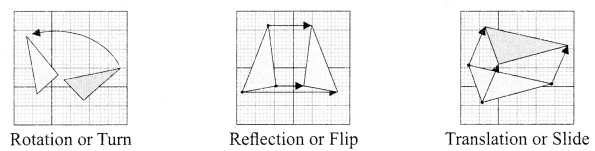If one shape can become another using resizing, then the shapes are similar.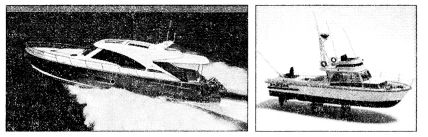Hence, two shapes are similar when one can become the other after a resize, flip, slide or turn.
(a) A model of a boat is made on the scale of 1 : 4. The model is 120 cm long. The full size of the boat has a width of 60 cm. What is the width of the scale model?
(i) 20 cm
(ii) 25 cm
(iii) 15 cm
(iv) 240 cm
Solution :
(iii) 15 cm

(b) What will effect the similarity of any two polygons?
(i) They are flipped horizontally.
(ii) They are dilated by a scale factor.
(iii) They are translated down.
(iv) They are not the mirror image of one another.
Solution :
(iv) They are not the mirror image of one another.

(c) If two similar triangles have a scale factor of a : b, which statement regarding the two triangles is true?
(i) The ratio of their perimeters is 3a : b
(ii) Their altitudes have a ratio a : b
(iii) Their medians have a ratio $$\frac{a}{2}: b$$
(iv) Their angle bisectors have a ratio a2: b2
Solution :
(ii) Their altitudes have a ratio a : b

(d) The shadow of a stick 5 m long is 2 m. At the same time, the shadow of a tree 12.5 m high is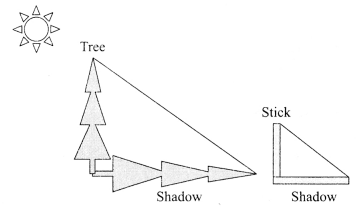(i) 3 m
(ii) 3.5 m
(iii) 4.5 m
(iv) 5 m
Solution :
(iv) 5 m

(e) Below you see a student’s mathematical model of a farmhouse roof with measurements. The attic floor, ABCD in the model, is a square. The beams that support the roof are the edges of a rectangular prism, EFGHKLMN. E is the middle of AT, F is the middle of BT, G is the middle of CT. and H is the middle of DT. All the edges of the pyramid in the model have length of 12 m.What is the length of EF, where EF is one of the horizontal edges of the block?
(i) 24 m
(ii) 3 m
(iii) 6 m
(iv) 10 m
Solution :
(iii) 6 m

Case Study Based-3

Question 19.
Applications of Parabolas-Highway Overpasses/Underpasses A highway underpass is parabolic in shape.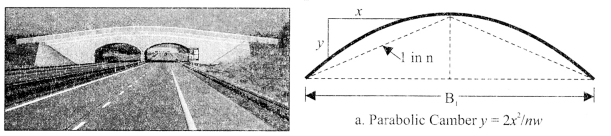Parabola: A parabola is the graph that results from p(x) — ax2 + bx + c. Parabolas are symmetric about a vertical line known as the Axis of Symmetry. The Axis of Symmetry runs through the maximum or minimum point of the parabola which is called the vertex.(a) If the highway overpass is represented by x2 – 2x – 8, then its zeroes are
(i) (2. -4)
(ii) (4, -2)
(iii) (-2, -2)
(iv) (-4, -4)
(ii) (4, -2)

(b) The highway overpass is represented graphically. Zeroes of a polynomial can be expressed graphically. Number of zeroes of polynomial is equal to number of points where the graph of polynomial
(i) intersects x-axis
(ii) intersects y-axis
(iii) intersects y-axis or x-axis
(iv) None of the above
(i) intersects x-axis

(c) Graph of a quadratic polynomial is a
(i) straight line.
(ii) Circle
(iii) Parabolla
(iv) ellipse
(iii) Parabolla

(d) The representation of Highway Underpass whose one zero is 6 and sum of the zeroes is 0, is 1
(i)  x2 – 6x + 2
(ii) x2 – 36
(iii) x2 – 6
(iv) x2 – 3
(ii) x2 – 36

(e) The number of zeroes that polynomial f(x) = (x – 2)2 + 4 can have is:
(i) 1
(ii) 2
(iii) 0
(iv) 3
(iii) 0

Case Study Based-4

Question 20.(a) Estimate the mean time taken by a student to finish the race.
(i) 54
(ii) 63
(iii) 43
(iv) 50
Solution :
(iii) 43

(b) What will be the upper limit of the modal class?
(i) 20
(ii) 40
(iii) 60
(iv) 80
Solution :
(iii) 60

(c) The construction of cumulative frequency table is useful in detennining the
(i) mean
(ii) median
(iii) mode
(iv) All of the above
Solution :
(ii) median

(d) The sum of lower limits of median class and modal class is
Solution :
(i) 60
(ii) 100
(iii) 80
(iv) 140
Solution :
(iii) 80

(e) How many students finished the race within 1 minute?
(i) 18
(ii) 37
(iii) 31
(iv) 8
Solution :
(iii) 31

Part-B
Section-III

All questions are compulsory. In case of internal choices, attempt anyone.

Question 21.
3 bells ring at an interval of 4, 7 and 14 minutes. All three bell rang at 6 am, when the three balls will the ring together next?
Solution :
4 = 2 x 2
7 =7 x 1
14 = 2 x 7
LCM = 2 x 2 x 7 = 28
The three bells will ring together again at 6 : 28 am

Question 22.
Find the point on x-axis which is equidistant from the points (2, -2) and (-4, 2).
OR
P(-2, 5) and Q(3, 2) are two points. Find the coordinates of the point R on PQ such that PR = 2QR
Solution: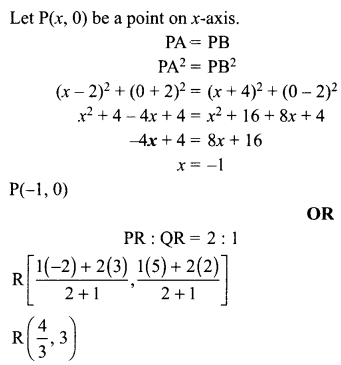Question 23.
Find a quadratic polynomial whose zeroes are $$5-3 \sqrt{2} \text { and } 5+3 \sqrt{2}$$
Solution: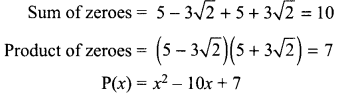Question 24.
Draw a line segment AB of length 9 cm. With A and B as centres, draw circles of radius 5 cm and 3 cm respectively. Construct tangents to each circle from the centre of the other circle.
Solution: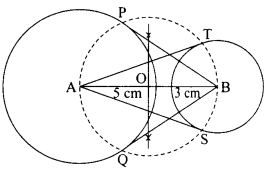Question 25.
If $$tan \mathrm{A}=\frac{3}{4}$$, find the value of $$\frac{1}{\sin A}+\frac{1}{\cos A}$$ and 3 cm respectively. Construct tangents to each circle from the centre of the other circle. If $$\sqrt{3} \sin \theta-\cos \theta=0 \text { and } 0^{\circ}<\theta<90^{\circ}$$ find the value of θ.
Solution: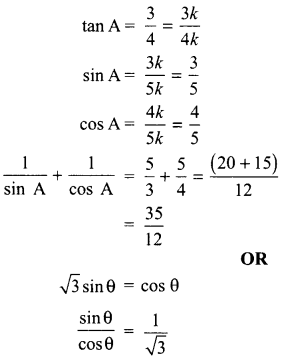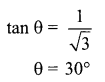Question 26.
In the figure, quadrilateral ABCD is circumscribing a circle with centre O and AD ⊥ AB. If radius of incircle is 10 cm, then find the value of x.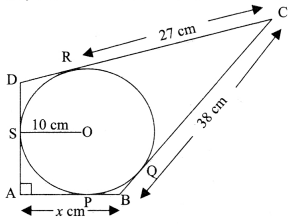Solution: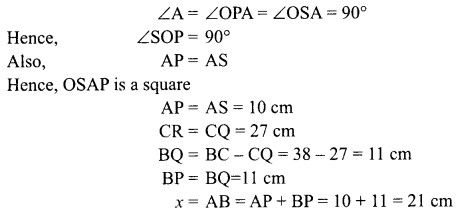Question 27.
Prove that 2 – √3 is irrational, given that √3 is irrational.
Solution: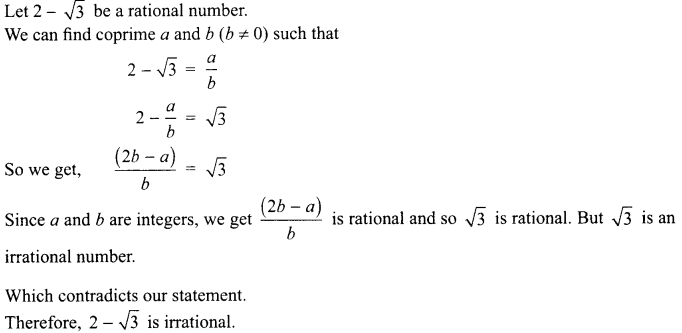Question 28.
If one root of the quadratic equation 3x2 + px + 4 = 0 is $$\frac{2}{3}$$, then find the value of p and the other root of the equation.
OR
The roots α and β of the quadratic equation x2 – 5x + 3(k – 1) = 0 are such that α – β = 1. Find the value k.
Solution :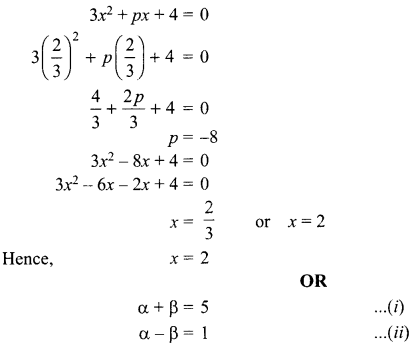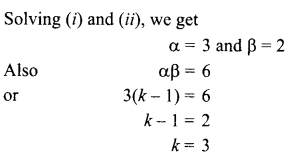Question 29.
In the figure, ABCD is a square of side 14 cm. Semicircles are drawn with each side of square as diameter. Find the area of the shaded region.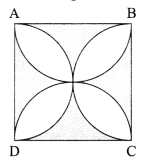Solution: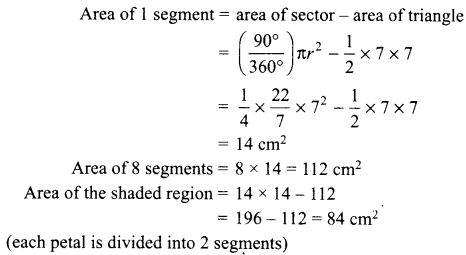Question 30.
The perimeters of two similar triangles are 25 cm and 15 cm respectively. If one side of the first triangle is 9 cm, find the length of the corresponding side of the second triangle.
OR
In an equilateral triangle ABC, D is a point on side BC such that BD = -BC. Prove that 9 AD2 = 7 AB2.
Solution: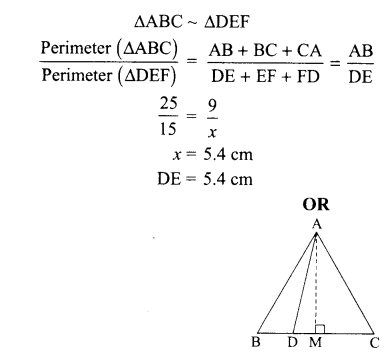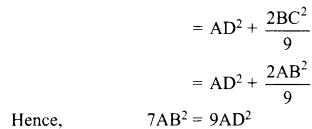Question 31.
The median of the following data is 16. Find the missing frequencies a and b, if the total of the frequencies is 70.

 Class 0-5 5-10 10-15 15-20 20-25 25-30 30-35 35-40 Frequency 12 Cl 12 15 b 6 6 4

Solution:

 Class Frequency Cumulative Frequency 0-5 12 12 5-10 a 12 + a 10-15 12 24 +a 15-20 15 39 + a 20-25 b 39 + a + b 25-30 6 45 + a + b 30-35 6 51+0 + 6 35-40 4 55 + o + 6 Total N = 70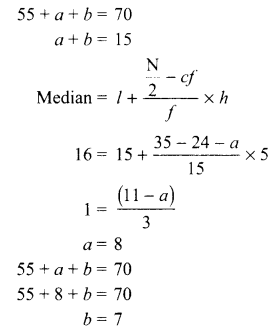Question 32.
If the angles of elevation of the top of the candle from two coins distant ‘a’ cm and ‘b’ cm (a > b) from its base and in the same straight line from it are 30° and 60°, then find the height of the candle.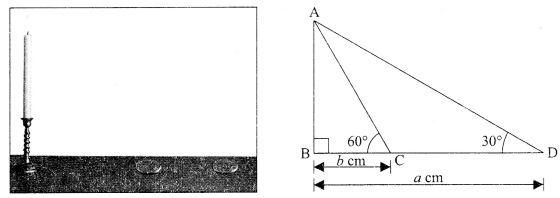Solution: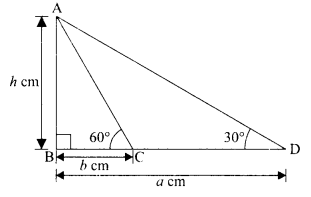Let AB = candle
C and D are two coins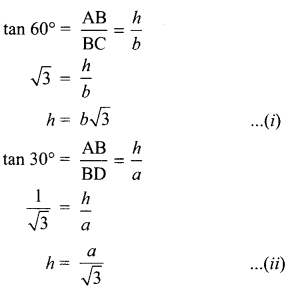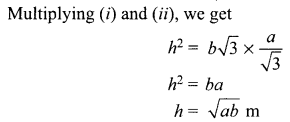Question 33.
The mode of the following data is 67. Find the missing frequency x:
Solution: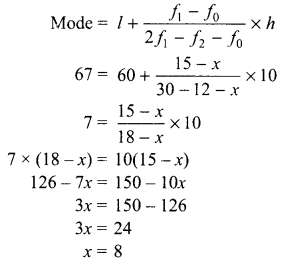Question 34.
The two palm trees are of equal heights and are standing opposite to each other on either side of the river, which is 80 m wide. From a point O between them on the river, the angles of elevation of the top of the trees are 60° and 30° respectively. Find the height of the trees and the distances of the point O from the trees.
OR
The angles of depression of the top and bottom of a building 50 metres high as observed from the top of a tower are 30° and 60° respectively. Find the height of the tower, and also the horizontal distance between the building and the tower.
Solution: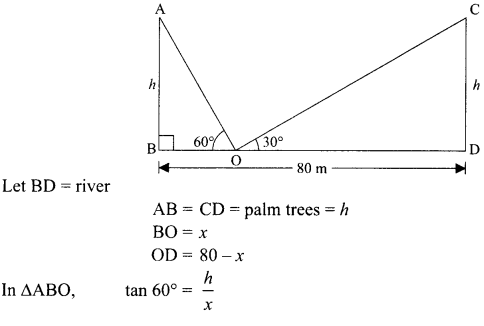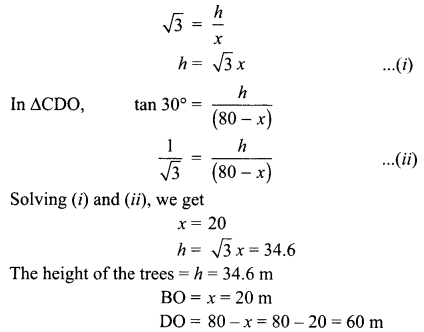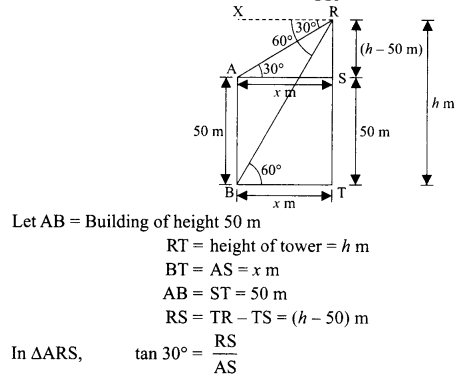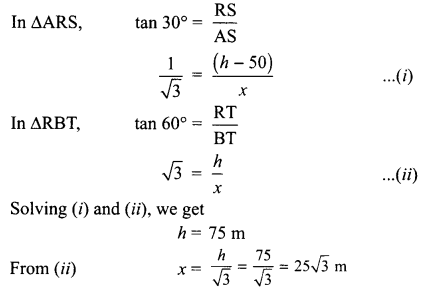Hence, height of the tower = h = 75 m
Distance between the building and the tower = 25 √3 = 43.25 m

Question 35.
Water is flowing through a cylindrical pipe of internal diameter 2 cm, into a cylindrical tank of base radius 40 cm at the rate of 0.7 m/sec. By how much will the water rise in the tank in half an hour?
Solution:
For pipe, r = 1cm
Length of water flowing in 1 sec, h = 0.1 m = 70 cm
Cylindrical Tank, R = 40 cm, rise in water level = H
Volume of water flowing in 1 sec = n×h = a × 1 x 1 × 70 = 70a
Volume of water flowing in 60 sec = 70a: x 60
Volume of water flowing in 30 minutes = 70a × 60 ×30
Volume of water in Tank = ar2H = a × 40 × 40 × H
Volume of water in Tank = Volume of water flowing in 30 minutes
a × 40 × 40 × H = 70a x 60 x 30

Question 36.
A motorboat covers a distance of 16 km upstream and 24 km downstream in 6 hours. In the same time, it covers a distance of 12 km upstream and 36 km downstream. Find the speed of the boat in still water and that of the stream.
Solution:
Let speed of the boat in still water = x km/hr,
and Speed of the stream = y km/hr
Downstream speed = (x + y) km/hr
Upstream speed = (x – y) km/hr 24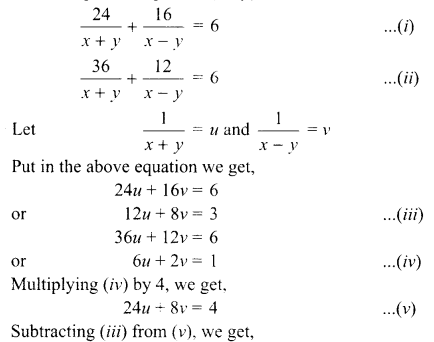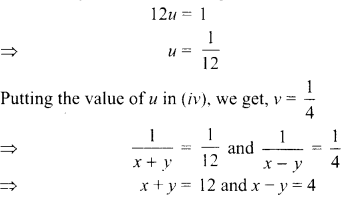Thus, speed of the boat in still water = 8 km/hr,
Speed of the stream = 4 km/hr